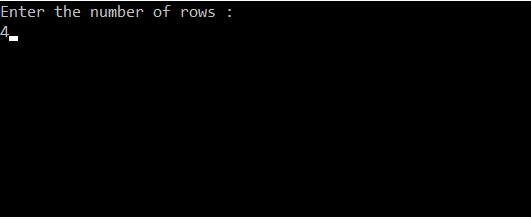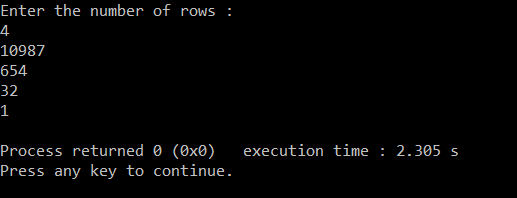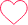# Inverted Right Triangle Number Printing

10987

654

32

1

## PREREQUISITE:

Basic knowledge of C language and use of loops.

## ALGORITHM:

1. Initialise an integer variable count=0 for finding the maximum number.
2. Take the number of rows as input from the user  and store it in any variable.(‘r‘ in this case).
3. Run a loop ‘r’ number of times to iterate through each of the rows. From i=r to i>0. The loop should be structured as for( i=r ; i>0 : i–)
4. Inside this loop calculate the maximum value of count by count+=i.
5. Run a loop ‘r’ number of times to iterate through each of the rows. From i=0 to i<r. The loop should be structured as for( i=0 ; i<r : i++).
6.  Run a nested loop inside the main loop to print the digits in each row of the triangle. From j=r to j>i. The loop should be structured as for( j=r; j>i ; j–).
7. In the nested loop print count and then decrement it.
8. In the main loop print a new line.

## Code in C:

```#include<stdio.h>
int main()
{
int i,j,r,count;                         //declaring integer variables i,j for loops , r for number of rows, count for decrement in value
count=0;                                //initialising count
printf("Enter the number of rows :\n"); //Asking user for input
scanf("%d",&r);                        //taking number of rows and saving it in variable r
for(i=r;i>0;i--)                       //loop to find the maximum value to print
{
count+=i;                        //incrementing count
}
for(i=0;i<r;i++)                       // loop for number of rows
{
for(j=r;j>i;j--)                 // loop for digits per each row
{
printf("%d",count);         //printing digit
count--;                    //decrementing count
}
printf("\n");                    // printing newline after each row
}
}```

## TAKING INPUT:DISPLAYING OUTPUT:### One comment on “Inverted Right Triangle Number Printing”

•Neerajsingh

//////////////////////////////////By @ neeraj singh chowhan///////////////////////////////////////////////////
n=4
d=”
c=n
b=int((n*(n+1))/2)
for i in range(0,n+1):
for j in range(0,c):
d=d+str(b)
b=b-1
print(d)
d=”
c=c-1
output:
10987
654
32
1
/////////////////////////////////////////// python code///////////////////////////////////////0